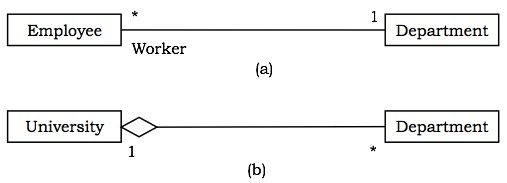# OOAD - UML Basic Notations

UML defines specific notations for each of the building blocks.

## Class

A class is represented by a rectangle having three sections −

• the top section containing the name of the class
• the middle section containing class attributes
• the bottom section representing operations of the class

The visibility of the attributes and operations can be represented in the following ways −

• Public − A public member is visible from anywhere in the system. In class diagram, it is prefixed by the symbol ‘+’.

• Private − A private member is visible only from within the class. It cannot be accessed from outside the class. A private member is prefixed by the symbol ‘−’.

• Protected − A protected member is visible from within the class and from the subclasses inherited from this class, but not from outside. It is prefixed by the symbol ‘#’.

An abstract class has the class name written in italics.

Example − Let us consider the Circle class introduced earlier. The attributes of Circle are x-coord, y-coord, and radius. The operations are findArea(), findCircumference(), and scale(). Let us assume that x-coord and y-coord are private data members, radius is a protected data member, and the member functions are public. The following figure gives the diagrammatic representation of the class.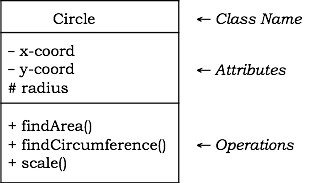## Object

An object is represented as a rectangle with two sections −

• The top section contains the name of the object with the name of the class or package of which it is an instance of. The name takes the following forms −

• object-name − class-name

• object-name − class-name :: package-name

• class-name − in case of anonymous objects

• The bottom section represents the values of the attributes. It takes the form attribute-name = value.

• Sometimes objects are represented using rounded rectangles.

Example − Let us consider an object of the class Circle named c1. We assume that the center of c1 is at (2, 3) and the radius of c1 is 5. The following figure depicts the object.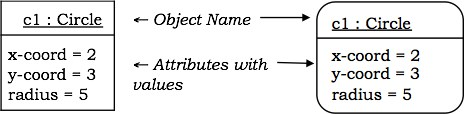## Component

A component is a physical and replaceable part of the system that conforms to and provides the realization of a set of interfaces. It represents the physical packaging of elements like classes and interfaces.

Notation − In UML diagrams, a component is represented by a rectangle with tabs as shown in the figure below.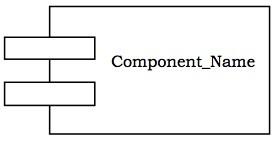## Interface

Interface is a collection of methods of a class or component. It specifies the set of services that may be provided by the class or component.

Notation − Generally, an interface is drawn as a circle together with its name. An interface is almost always attached to the class or component that realizes it. The following figure gives the notation of an interface.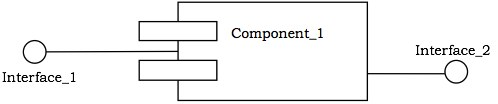## Package

A package is an organized group of elements. A package may contain structural things like classes, components, and other packages in it.

Notation − Graphically, a package is represented by a tabbed folder. A package is generally drawn with only its name. However it may have additional details about the contents of the package. See the following figures.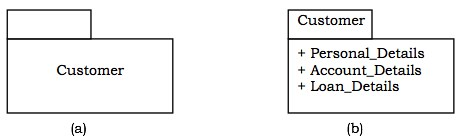## Relationship

The notations for the different types of relationships are as follows −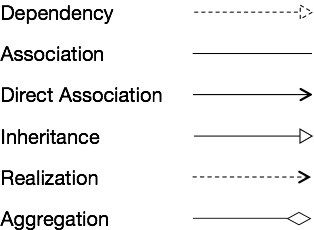Usually, elements in a relationship play specific roles in the relationship. A role name signifies the behavior of an element participating in a certain context.

Example − The following figures show examples of different relationships between classes. The first figure shows an association between two classes, Department and Employee, wherein a department may have a number of employees working in it. Worker is the role name. The ‘1’ alongside Department and ‘*’ alongside Employee depict that the cardinality ratio is one–to–many. The second figure portrays the aggregation relationship, a University is the “whole–of” many Departments.